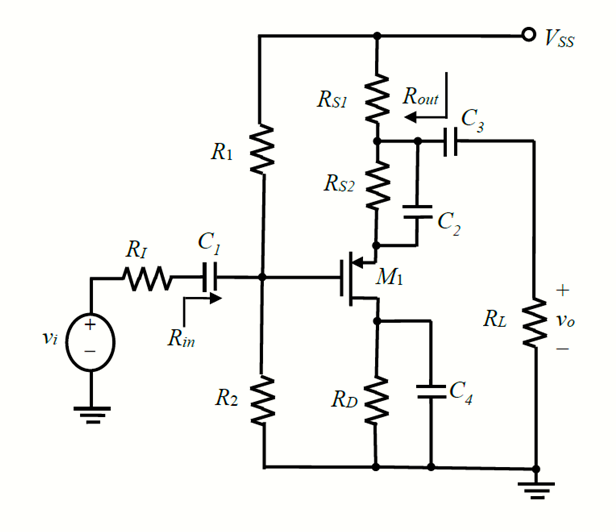UP TO 15 % DISCOUNT

Get Your Assignment Completed At Lower Prices

Plagiarism Free Solutions
100% Original Work
24*7 Online Assistance
Native PhD Experts
Hire a Writer Now
ENG101: Analogue Electronics Design Assignment, SUSS, Singapore Draw the DC equivalent circuit and Calculate the Q-point of the transistor
 University Singapore University of Social Science (SUSS) Subject ENG101: Analogue Electronics Design
Posted on: 4th Aug 2023

# ENG101: Analogue Electronics Design Assignment, SUSS, Singapore Draw the DC equivalent circuit and Calculate the Q-point of the transistor

In Figure 1, the MI has lip = 1 mA/V2, VTP = -1 V and = 0.015 V’. Assume that all the capacitors have infinite value, R./ = 50 0, RI = 2 MO, R2 = 0.5 Mcl, RD= 2 kO, Rst = 9 K2, Rs2 = 1 In, RI= 100 K2 and Vss = 15 V. When calculating ID for Mi in saturation region, can ignore the channel length modulation: ./D = ip(I VGSI IVTPD2

(a) Draw the DC equivalent circuit.

(b) Calculate the Q-point of the transistor Mi in Figure 1.

(c) Determine if the transistor Mi is operating in the Saturation region.

(d) Calculate the DC power dissipation of each component of the circuit.

(e) Verify if the total DC power dissipated by the circuit computed in (d) is equal to the total power delivered by Vss

(f) Draw a small-signal equivalent circuit of the amplifier.

(g) Calculate the resistances Rim, Rout of the amplifier.

(h) If vi= 0.1sintut mV, what will be the output voltage vo?

(i) Calculate the current gain Ig

(j) What is the maximum amplitude of the input signal vt for this small-signal analysis to be valid? Will the output waveform of (h) be distorted?

(k) What will be the value of R. to maximize the voltage gain v, of this amplifier?

(1) Without changing the transistor, suggest two effective ways to increase the voltage gain of this amplifier circuit. Please indicate the direction of change if your suggestions involve changes in component values.Get Help By Expert

"Enhance Your Analog Electronics Design Skills with Expert Online Assignment Assistance" Dive into the world of analog electronics design through personalized guidance and support from My Assignment Help SG. Elevate your understanding at the Singapore University of Social Science (SUSS) as you tackle assignments with confidence. Unveil innovative approaches, receive step-by-step solutions, and master circuitry intricacies. Unleash your potential today!

Categories:-
Tags:- ENG101 SUSS SUSS TMA##### No Need To Pay Extra
• Turnitin Report

\$10.00

\$9.00
Per Page
• Consultation with Expert

\$35.00
Per Hour
• Live Session 1-on-1

\$40.00
Per 30 min.
• Quality Check

\$25.00
##### Free

New Special Offer

## Get 30% Off

UP TO 15 % DISCOUNT

Get Your Assignment Completed At Lower Prices

Plagiarism Free Solutions
100% Original Work
24*7 Online Assistance
Native PhD Experts
Hire a Writer Now
My Assignment Help SG Services
My Assignment Help SG